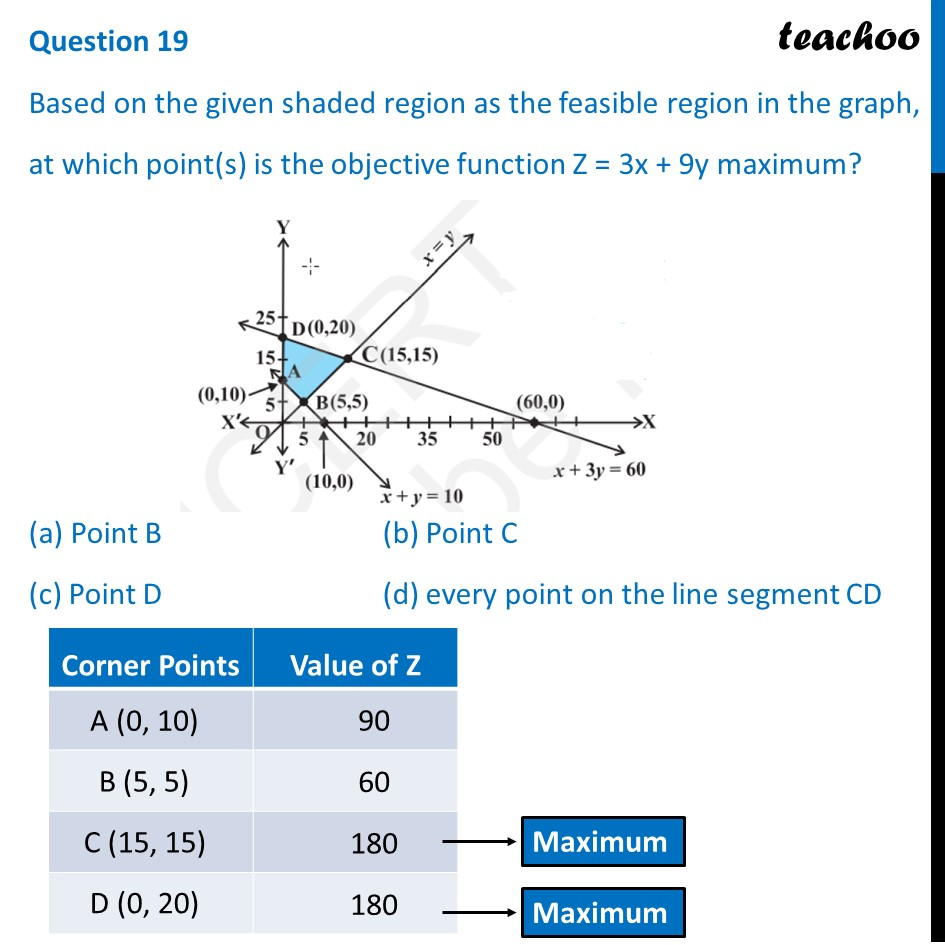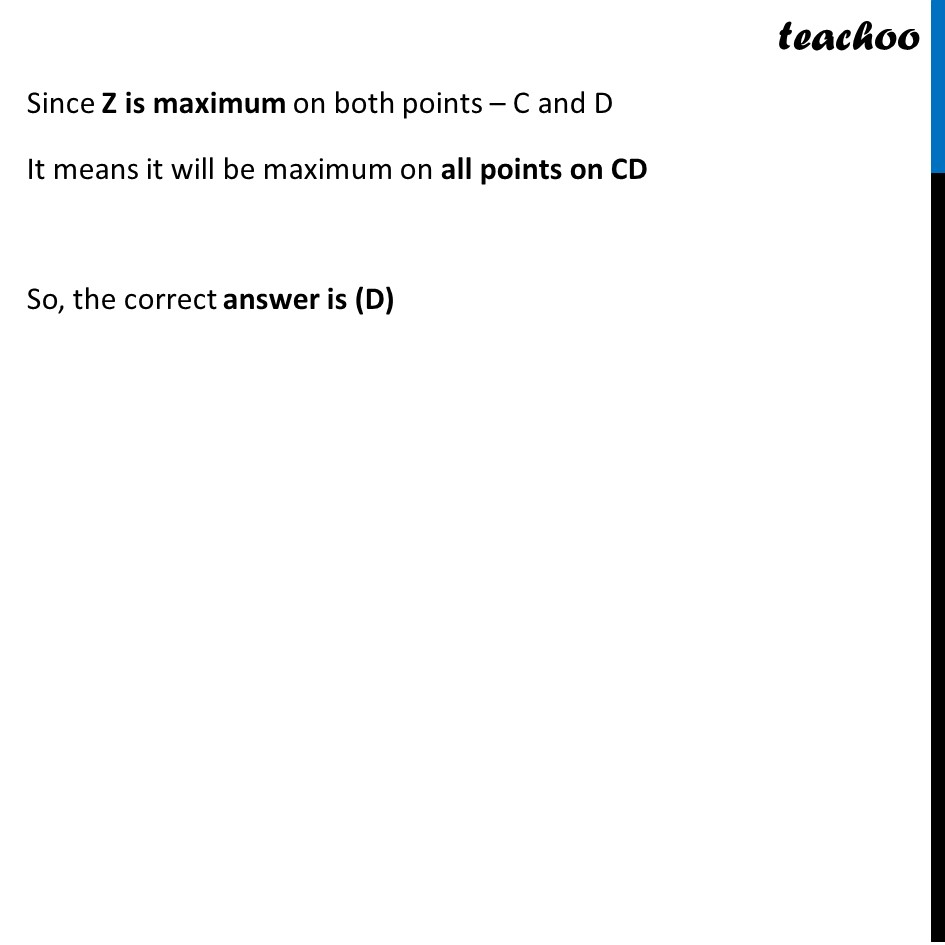CBSE Class 12 Sample Paper for 2022 Boards (MCQ Based - for Term 1)

Class 12
Solutions of Sample Papers and Past Year Papers - for Class 12 Boards

## (a) Point B  (b) Point C (c) Point D  (d) every point on the line segment CD### Transcript

Based on the given shaded region as the feasible region in the graph, at which point(s) is the objective function Z = 3x + 9y maximum? (a) Point B (b) Point C (c) Point D (d) every point on the line segment CD Since Z is maximum on both points – C and D It means it will be maximum on all points on CD So, the correct answer is (D)